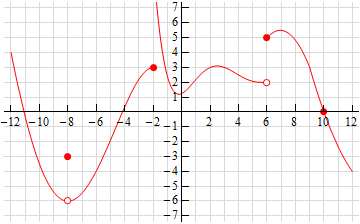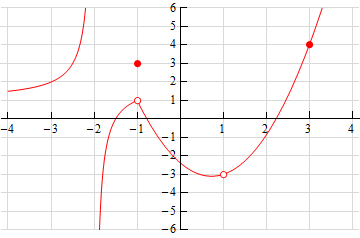Paul's Online Notes
Home / Calculus I / Limits / The Limit
Show Mobile Notice Show All Notes Hide All Notes
Mobile Notice
You appear to be on a device with a "narrow" screen width (i.e. you are probably on a mobile phone). Due to the nature of the mathematics on this site it is best views in landscape mode. If your device is not in landscape mode many of the equations will run off the side of your device (should be able to scroll to see them) and some of the menu items will be cut off due to the narrow screen width.

### Section 2.2 : The Limit

1. For the function $$\displaystyle f\left( x \right) = \frac{{8 - {x^3}}}{{{x^2} - 4}}$$ answer each of the following questions.
1. Evaluate the function at the following values of $$x$$ compute (accurate to at least 8 decimal places).
1. 2.5
2. 2.1
3. 2.01
4. 2.001
5. 2.0001
1. 1.5
2. 1.9
3. 1.99
4. 1.999
5. 1.9999
2. Use the information from (a) to estimate the value of $$\displaystyle \mathop {\lim }\limits_{x \to 2} \frac{{8 - {x^3}}}{{{x^2} - 4}}$$.
Solution
2. For the function $$\displaystyle R\left( t \right) = \frac{{2 - \sqrt {{t^2} + 3} }}{{t + 1}}$$ answer each of the following questions.
1. Evaluate the function at the following values of $$t$$ compute (accurate to at least 8 decimal places).
1. -0.5
2. -0.9
3. -0.99
4. -0.999
5. -0.9999
1. -1.5
2. -1.1
3. -1.01
4. -1.001
5. -1.0001
2. Use the information from (a) to estimate the value of $$\displaystyle \mathop {\lim }\limits_{t \to \, - 1} \frac{{2 - \sqrt {{t^2} + 3} }}{{t + 1}}$$.
Solution
3. For the function $$\displaystyle g\left( \theta\right) = \frac{{\sin \left( {7\theta } \right)}}{\theta }$$ answer each of the following questions.
1. Evaluate the function at the following values of $$\theta$$ compute (accurate to at least 8 decimal places). Make sure your calculator is set to radians for the computations.
1. 0.5
2. 0.1
3. 0.01
4. 0.001
5. 0.0001
1. -0.5
2. -0.1
3. -0.01
4. -0.001
5. -0.0001
2. Use the information from (a) to estimate the value of $$\displaystyle \mathop {\lim }\limits_{\theta\to \,0} \frac{{\sin \left( {7\theta } \right)}}{\theta }$$.
Solution
4. Below is the graph of $$f\left( x \right)$$. For each of the given points determine the value of $$f\left( a \right)$$ and $$\mathop {\lim }\limits_{x \to a} f\left( x \right)$$. If any of the quantities do not exist clearly explain why.
1. $$a = - 3$$
2. $$a = - 1$$
3. $$a = 2$$
4. $$a = 4$$
Solution5. Below is the graph of $$f\left( x \right)$$. For each of the given points determine the value of $$f\left( a \right)$$ and $$\mathop {\lim }\limits_{x \to a} f\left( x \right)$$. If any of the quantities do not exist clearly explain why.
1. $$a = - 8$$
2. $$a = - 2$$
3. $$a = 6$$
4. $$a = 10$$
Solution6. Below is the graph of $$f\left( x \right)$$. For each of the given points determine the value of $$f\left( a \right)$$ and $$\mathop {\lim }\limits_{x \to a} f\left( x \right)$$. If any of the quantities do not exist clearly explain why.
1. $$a = - 2$$
2. $$a = - 1$$
3. $$a = 1$$
4. $$a = 3$$
Solution